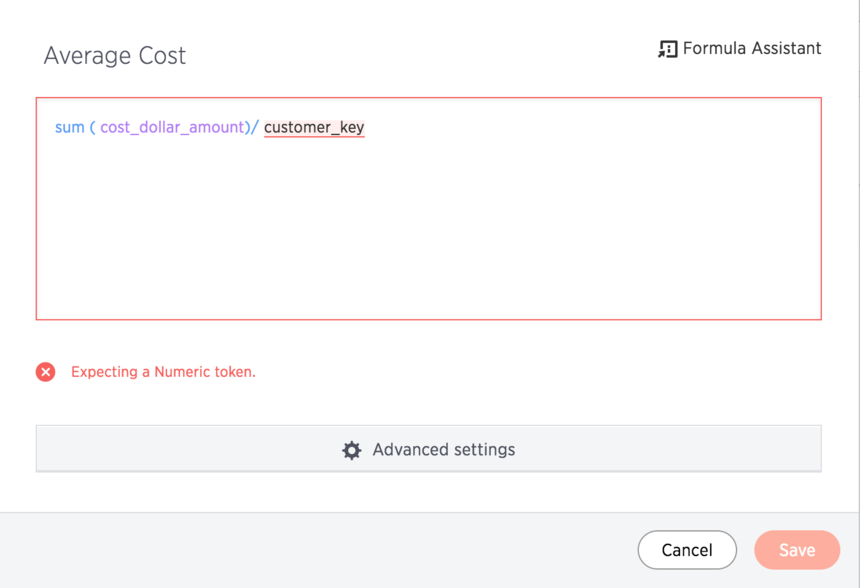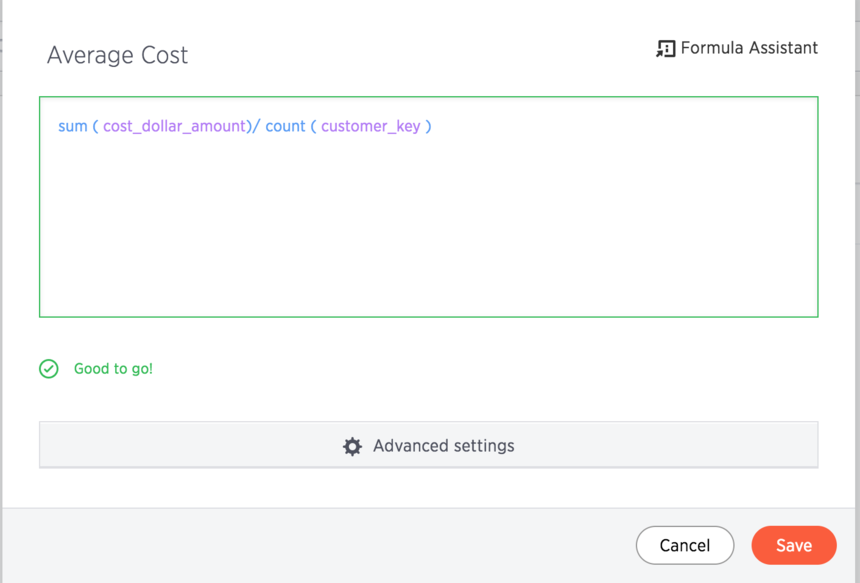# Trying to combine row level and aggregate formulae

Why are row level and aggregate formulas not accepted when combined? I tried to save sum ( cost_dollar_amount)/  customer_key , but it throws an error.

2replies Oldest first
• Oldest first
• Popular•The formula above contains a mixture of row level and aggregate formulae in a single expression which is not allowed in ThoughtSpot. The cost_dollar_amount is an aggregate expression value whereas the customer_key is a row level formula. You can get the desired result by using the formula ‘sum ( cost_dollar_amount)/ count (customer_key) ’.

Incorrect formula-Correct Formula-Like
•As for the reasons as to why you should not combine them, once you aggregate the data, you lose the values at the individual row level and hence cannot access them.

Let us take the following example

Class Name             Total Marks           Class Strength

*************************************************************

Economics               350                       8

Math                         700                      12

Physics                     600                      9

Let's assume I have a formula as sum(Total Marks) / Class Strength. To calculate the sum, I have aggregated across all the rows and don't have access to the individual rows like 8, 12 and 9 and to be frank that formula doesn't make much sense either. So the formula will have to be corrected to Sum(Total Marks)/ Sum(Class Strength)

Like
Like Follow